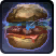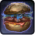# NomConditions

Used by

## Related effects, buffs and debuffs

• [1.8s]Nom
Going in for a snack!

 Slot: Buff Duration: 1.8s Tick rate: 0.2s # occurrences: 0 Tags: tag.​abl.​operation.​generic.​ability_delay.​ability_1 Target overrides: [target override] Aoe Sphere
• On Apply

Perform the following actions:

• Immobilize and prevent rotation
• Unknown (183)
- Unknown (303) = (bool) true
- Unknown (306) = (bool) false
- Unknown (430) = (bool) false
- Unknown (310) = (string) aoePositionOptionType_Entity
- Unknown (311) = (string) aoeTarget_Ground
- Unknown (313) = (string) aoeScalarType_LiteralValue
- Unknown (317) = (string) aoeScalarType_LiteralValue
- Unknown (321) = (string) aoeScalarType_LiteralValue
- Unknown (325) = (string) aoeOrientationOptionType_WorldCoordinates
- Unknown (344) = (string) aoeSphere
- Unknown (349) = (string) aoeTargetCondition_IsPlayer;aoeTargetCondition_IsAlive
- Unknown (353) = (string) aoeScalarType_LiteralValue
- Unknown (354) = (string) aoeScalarType_LiteralValue
- Unknown (355) = (string) aoeScalarType_LiteralValue
- Unknown (367) = (string) aoeScalarType_LiteralValue
- Unknown (384) = (string)
- Unknown (386) = (string) aoeAbilityCaster_Caster
- Unknown (387) = (string) aoeAbilityTarget_Target
- Unknown (388) = (string) operations/dxun/boss_4/crab_nom_groundwarning
- Unknown (431) = (string) aoeRotation_None
- Unknown (541) = (string) aoeVfxEntity_AreaEffect
- Unknown (551) = (string) aoeVfx_PlayAtPosition
- Ability Spec = (int) 16141065782512003872
- Unknown (307) = (int) 1
- Unknown (308) = (int) 1
- Tick Rate = (float) 1.8
- Unknown (304) = (float) 1.8
- Unknown (305) = (float) 1.8
- Unknown (314) = (float) 0
- Unknown (318) = (float) 0
- Unknown (322) = (float) 0
- Unknown (362) = (float) 0
- Unknown (363) = (float) 0
- Unknown (364) = (float) 0
- Unknown (368) = (float) 0.2
- Unknown (390) = (float) 0
- Unknown (398) = (float) 1.8
• When effect ticks the 4th time

Perform the following actions:

• Play appearance epp . operation . dxun . boss . huntmaster . beasts . crab . nom_instant, dependent on calling effect